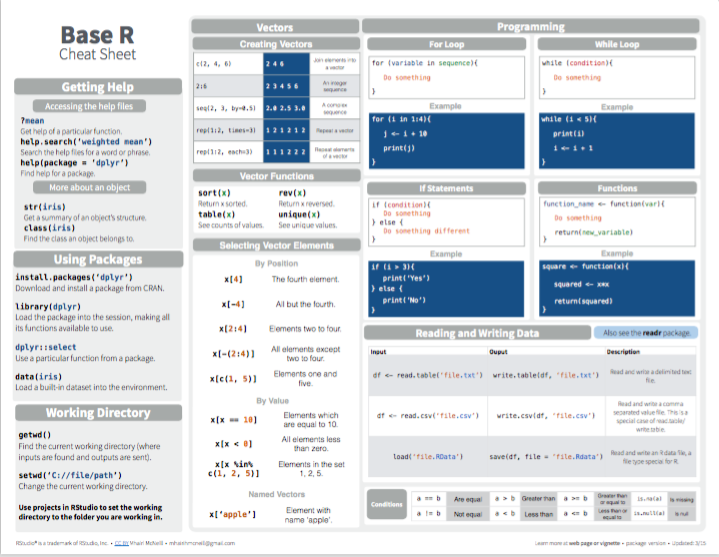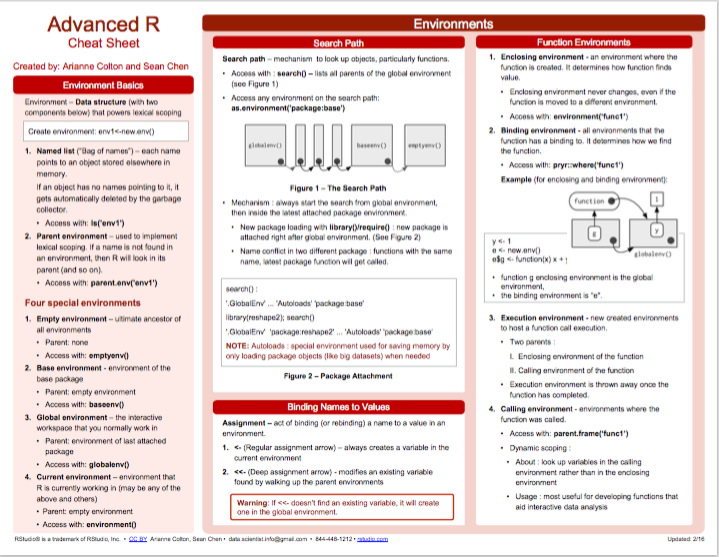## 3.6 Conclusion

### 3.6.1 Summary

The previous and this chapter provided a very brief introduction to R . Conceptually, we introduced and distinguished between

• different types of R objects (e.g., data vs. functions),
• different types of data (e.g., logical, numeric, and character data), and
• different data structures and shapes of data (e.g., linear vs. rectangular vectors or lists).

In R, a range of different objects are created and changed by applying functions (e.g., for assignment, arithmetic, and many other operations). Thus, the following chapters gravitate around creating more objects and using a large variety of functions to do things with them.

### 3.6.2 ResourcesThere is no shortage of introductory books and scripts on R, but it is helpful to look for one that fits your interests and level of expertise.

#### Books and online scripts

As most introductions of R focus on vectors and data frames, they provide only scarce information on other data structures. Some noteworthy exceptions (with dedicated chapters or sections on lists and factors) are:

• Norman Matloff’s The art of R programming

• Hadley Wicham’s and Garrett Grolemund’s textbook R for Data Science (r4ds)

#### Cheatsheets

Here are some pointers to related RStudio cheatsheets:

• Base R:Figure 3.1: Base R summary from RStudio cheatsheets.#### Miscellaneous

Other helpful links that do not fit into the above categories include:

• R-bloggers collects blog posts on R.

• R-exercises provides categorized sets of exercises to help people developing their R programming skills.

• Quick-R (by Robert Kabacoff) is a popular website on R programming.

### 3.6.3 Preview

In Chapter 4, we will learn to use R functions for visualizing data.

### References

Matloff, N. (2011). The art of R programming: A tour of statistical software design. No Starch Press.
R Core Team. (2021). R base: A language and environment for statistical computing. R Foundation for Statistical Computing. https://www.R-project.org